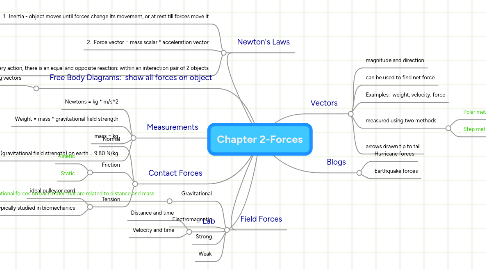# Chapter 2-Forces

Get Started. It's FreeChapter 2-Forces## 1. Vectors

### 1.4. measured using two methods

1.4.1. Polar method: descriptive, vector arrow with angle to horizontal

1.4.2. Step method: x hat and y hat, make triangle of x and y components, use SOH CAH TOA

## 3. Newton's Laws

### 3.1. 1. Inertia - object moves until forces change its movement, or at rest till forces move it

3.1.1. Equilibrium

3.1.1.1. moving at constant speed and direction

3.1.1.2. staying at rest

### 3.3. 3. For every action, there is an equal and opposite reaction; within an interaction pair of 2 objects

3.3.1. Internal forces

3.3.2. External forces

## 4. Field Forces

### 4.1. Gravitational

4.1.1. Newton's Law: any two objects exert gravitational forces on each other that are related to distance and mass

4.1.1.1. F = G*m1*m2 / r^2

4.1.1.2. G = universal gravitational constant

## 5. Contact Forces

5.2.1. Kinetic

5.2.2. Static

### 5.3. Tension

5.3.1. ideal pulley or cord

5.3.2. typically studied in biomechanics